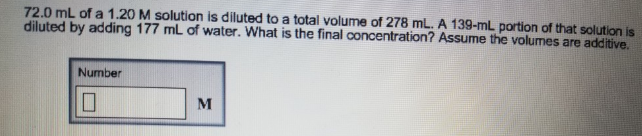# Problem: 72.0 mL of a 1.20 M solution is diluted to a total volume of 278 mL. A 139-mL portion of that solution is diluted by adding 177 mL of water. What is the final concentration? Assume the volumes are additive.

###### FREE Expert Solution
99% (109 ratings)###### Problem Details

72.0 mL of a 1.20 M solution is diluted to a total volume of 278 mL. A 139-mL portion of that solution is diluted by adding 177 mL of water. What is the final concentration? Assume the volumes are additive.